# Algebra II : Normal Distributions

## Example Questions

### Example Question #421 : Basic Statistics

The ages of the students at GW High School are normally distributed with a mean ofand a standard deviation ofyears.

What is the proportion of students that are younger thanyears old?

Not enough information to answer the question.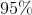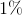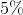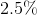Explanation:

This question relates to the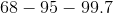rule of normal distribution. We know thatof the data are withinstandard deviations from the mean.

In this case this means thatof the students are between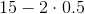and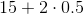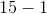and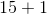andTherefore we haveof the students that are outside of this range. Since the normal distribution is symmetric, the proportion of students belowis the same as the proportion of students above.

Thus the right answer is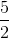or.

### Example Question #1 : Graphing Data

The scores for your recent english test follow a normal distribution pattern. The mean was a 75 and the standard deviation was 4 points. What percentage of the scores were below a 67?

10%

2.5%

5%

7.5%

2.5%

Explanation:

Use the 68-95-99.7 rule which states that 68% of the data is within 1 standard deviation (in either direction) of the mean, 95% is within 2 standard deviations, and 99.7% is within 3 standard deviations of the mean.

In this case, 95% of the students' scores were between:

75-(2 x 4) and 75+(2 x 4)

or between a 67 and a 83, with equal amounts of the leftover 5% of scores above and below those scores. This would mean that 2.5% of the students scored below a 67% on the test.

### Example Question #1 : Normal Distributions

Your class just took a math test. The mean test score was a 78 with a standard deviation of 2 points. With this being the case, 99.7% of the class scored between what two scores?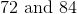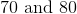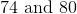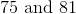Explanation:

Use the 68-95-99.7 rule which states that 68% of the data is within 1 standard deviation (in either direction) of the mean, 95% is within 2 standard deviations, and 99.7% is within 3 standard deviations (in either direction) of the mean.

In this case, 99.7% of the students' scores were between 3 standard deviation above the mean and 3 standard deviations below the mean:

78-(2 x 3) and 78+(2 x 3)

or between a 72 and an 84.

### Example Question #4 : Normal Distributions

All of the following statements regarding a Normal Distribution are true except:

The shape of the graph of a normally-distributed data set is dependent upon the mean and the standard deviation of the data set that it describes.

A graph of a normally-distributed data set is symmetrical.

A graph of a normally-distributed data set will have a single, central peak at the mean of the data set that it describes.

Between two graphs of normally-distributed data sets, the graph of the set with a higher standard deviation will be wider than the graph of the set with a lower standard deviation.

All of these are true.

All of these are true.

Explanation:

The graph of a normally-distributed data set is symmetrical.

The graph of a normally-distributed data set has a single, central peak at the mean of the data set that it describes.

The graph of a normally-distributed data set will vary based only upon the mean and the standard deviation of the set that it describes.

The graph of a normally-distributed data set with a higher standard deviation will be wider than the graph of a normally-distributed data set with a lower standard deviation.

The question asks us to find the statement that is not true; however, all statements are true so the correct response is "All of these are true."

### All Algebra II Resources